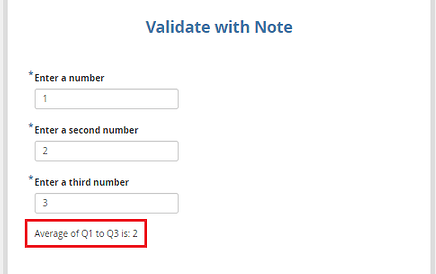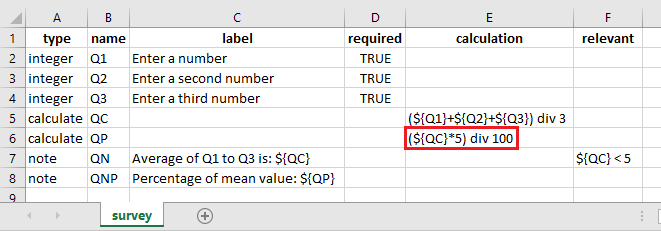# Calculating percentage in KoBoToolbox

Hi,

Thanks for the reply, it is working fine.
Can we calculate the percentage of that Average value.
For Example:
Average of the three vaules is 2
Percentage: 2*5% = 0.05
finally we need to display 0.05 value.

Thanks!

Hi @keerthi,

Could you please explain on how you calculated the percentage so that we could help you on how it looks in the xlsform.

Have a great day!

Hi

Q1: Enter a number
Q2: Enter second number
Q3: Enter Third Number
Q4: Mean value **\we have calculated the mean value and displayed as 2
Q5: Percentage of mean value **\ as per the below screenshot the average we have got is 2 so 2*5% = 0.05 (2 multiplied with 5% = 0.05) we only need to display 0.05 in the Q5
**Thanks!

Hi @keerthi,

You could do the same as outlined in the image below:

In the survey tab of your xlsform:Data entry screen as seen in Enketo:

Reference xlsform:
Validate with Note.xlsx (10.4 KB)